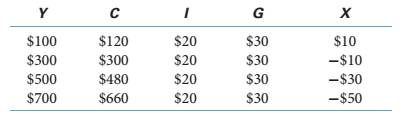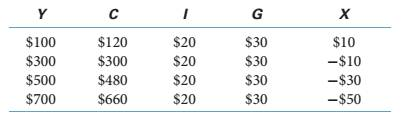Chapter 10, Problem 15E

Chapter
Section
Textbook Problem

Use the information in the following table to do exercises 8-15:What are the equations for the consumption, net exports, and aggregate expenditures functions?

To determine

Use the information in the following table:What are the equations for the consumption, net exports and aggregate expenditures functions?

Explanation

Given the data as follows

Consumption function is calculated as

C=Ca+cY

We have Y=500, C=480 and MPC=0.9

Substituting the values in above formula, we get

480=Ca+(0.9×500)

480=Ca+450

Ca=480450

Ca=30

Autonomous consumption is constant at every level of income.

Thus, consumption function is given as

C=30+0

Still sussing out bartleby?

Check out a sample textbook solution.

See a sample solution

The Solution to Your Study Problems

Bartleby provides explanations to thousands of textbook problems written by our experts, many with advanced degrees!

Get Started

Find more solutions based on key concepts